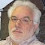# N-bit ring counter in VHDL

## This VHDL project is to implement a parameterized N-bit switch tail ring counter using VHDL. VHDL code for parameterized ring counter is presented in this project.

#### It means that users can easily change the number of bits of the ring counter without modifying VHDL code inside the ring counter. There is a parameter N to define the number of bits of the ring counter, and when we want to change the number of bits, just change the parameter N and re-synthesize or simulate.#### VHDL code for D Flip-Flop:

```library IEEE;
use IEEE.STD_LOGIC_1164.ALL;
-- fpga4student.com FPGA projects, VHDL projects, Verilog projects
-- VHDL project: VHDL code for ring counter
-- VHDL code for DFF
entity DFF is
port(
Q : out std_logic;      -- Data output
CLK :in std_logic;      -- Clock input
RESET :in std_logic;  -- Synchronous reset input
D :in  std_logic      -- Data input
);
end DFF;
architecture Behavioral of DFF is  --architecture of the circuit.
begin  --"begin" statement for architecture.
process(CLK,RESET) --process with sensitivity list.
begin  --"begin" statment for the process.
if (RESET = '1') then-- asynchronous reset
Q <= '0';
elsif( rising_edge(CLK) ) then
Q <= D;
end if;
end process;  --end of process statement.
end Behavioral;
```

The above DFF has one asynchronous reset pin which is to reset the state of the DFF at anytime. It means that it does not need to wait for the rising edge of the clock.

### VHDL code for ring counter using behavioral modelings:

```library IEEE;
use IEEE.STD_LOGIC_1164.ALL;
------------------------------------------
---- N bit switch tail ring counter ------
------------------------------------------
-- Behavior
-- fpga4student.com FPGA projects, VHDL projects, Verilog projects
-- VHDL project: VHDL code for ring counter
entity Behavior_Ring_Counter is
generic ( N : integer:=4 );
port (
CLK: in std_logic; -- clock
RESET: in std_logic; -- reset
Q_OUT: out std_logic_vector(N-1 downto 0) -- output

);
end Behavior_Ring_Counter;
-- fpga4student.com FPGA projects, VHDL projects, Verilog projects
architecture Behavioral of Behavior_Ring_Counter is
signal not_QN: std_logic;
signal Q : std_logic_vector(N-1 downto 0):=(others => '0');
begin
not_QN <= not Q(N-1);
process(CLK,RESET)
begin
if(RESET='1') then -- asynchronous reset
Q <= (others => '0');
elsif(rising_edge(CLK)) then
Q <= Q(N-2 downto 0) & not_QN; -- Switch TAil ring counter
end if;
end process;
Q_OUT <= Q;
end Behavioral;
```

### VHDL code for ring counter using structural modelings:

```-- fpga4student.com FPGA projects, VHDL projects, Verilog projects
-- VHDL project: VHDL code for ring counter
library IEEE;
use IEEE.STD_LOGIC_1164.ALL;
------------------------------------------
---- N bit switch tail ring counter ------
------------------------------------------
-- STructurally
entity Structural_Ring_Counter is
generic ( N : integer:=4 );
port (
CLK: in std_logic; -- clock
RESET: in std_logic; -- reset
Q_OUT: out std_logic_vector(N-1 downto 0) -- output

);
end Structural_Ring_Counter;

architecture structural of Structural_Ring_Counter is
-- fpga4student.com FPGA projects, VHDL projects, Verilog projects
signal not_QN: std_logic;
signal D,Q : std_logic_vector(N-1 downto 0):=(others => '0');
begin
-- fpga4student.com FPGA projects, VHDL projects, Verilog projects
N_BIT_Ring_counter : for i in 0 to N-1 generate
begin
F0 : if ( i = 0 ) generate   -- First DFF
begin U1 : entity work.DFF port map
(
Q => Q(0),
CLK => CLK,
RESET => RESET,
D => not_QN
);
end generate F0;
F1 : if ( i /= 0 ) generate  -- The rest of DFFs
begin U2 : entity work.DFF port map
(
Q => Q(i),
CLK => CLK,
RESET => RESET,
D => Q(i-1)
);
end generate F1;
end generate N_BIT_Ring_counter;
not_QN <= not Q(N-1);
Q_OUT <= Q;
end structural;
```

A VHDL testbench is also provided to verify the operation of the above code.

#### VHDL testbench code for ring counter:

```LIBRARY ieee;
USE ieee.std_logic_1164.ALL;
-- fpga4student.com FPGA projects, VHDL projects, Verilog projects
-- VHDL project: VHDL code for ring counter
-- VHDL Testbench code for ring counter
ENTITY tb_counter IS
END tb_counter;

ARCHITECTURE behavior OF tb_counter IS

-- Component Declaration for the Unit Under Test (UUT)

COMPONENT Structural_Ring_Counter
GENERIC
( N : integer:=5);
PORT(
CLK : IN  std_logic;
RESET : IN  std_logic;
Q_OUT : OUT  std_logic_vector(4 downto 0)
);
END COMPONENT;

--Inputs
signal CLK : std_logic := '0';
signal RESET : std_logic := '0';

--Outputs
signal Q_OUT : std_logic_vector(4 downto 0);

-- Clock period definitions
constant CLK_period : time := 10 ns;

BEGIN
-- fpga4student.com FPGA projects, VHDL projects, Verilog projects
-- Instantiate the Unit Under Test (UUT)
uut: Structural_Ring_Counter
generic map
(
N => 5-- change number of bits here
)
PORT MAP (
CLK => CLK,
RESET => RESET,
Q_OUT => Q_OUT
);

-- Clock process definitions
CLK_process :process
begin
CLK <= '0';
wait for CLK_period/2;
CLK <= '1';
wait for CLK_period/2;
end process;

-- Stimulus process
stim_proc: process
begin
-- hold reset state for 100 ns.
RESET <= '1';
wait for 100 ns;
RESET <= '0';
wait for CLK_period*10;

-- insert stimulus here

wait;
end process;

END;
```

As we can see from the testbench, to change the number of bits of the ring counter, just modify the input parameter N and re-simulate.

#### Simulation result for the ring counter:#### Both behavior and structural VHDL code are synthesizable. Below are the RTL Schematic for the behavioral modeling ring counter.1.2.